Using JMP > Create Formulas in JMP > Order Expressions in Formulas > Build a Formula in Order of Precedence
Publication date: 11/29/2021

## Build a Formula in Order of Precedence

It is best to build a formula starting with any expression that serves as an argument. This is because functions have a high order of precedence and are always grouped with their corresponding arguments. It is also a good idea to create expressions working from highest to lowest order of precedence when possible. If you need parentheses, be sure to enter the open parenthesis before entering the expression to be enclosed.

For example, given a data table with the columns A, B, and C, use the following steps to compose the expression A(B + C). Note that this expression is not the same as A x B + C, which evaluates as (A x B) + C.

To enter the expression, follow these steps:

1. Click column A in the Columns list.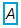2. Click the multiplication button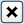in the Formula Editor keypad.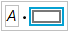3. Enter an open parenthesis.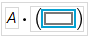4. Click column B in the Columns list.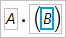5. Click the addition button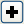in the Formula Editor keypad.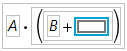6. Click column C in the Columns list.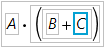Because the order of precedence determines which arguments are affected by each functions, it also affects the grouping of expressions. Select functions in the formula to verify how the order-of-precedence rules have been applied.

### Structure Formulas for Efficient Evaluation

Usually, it is not necessary to structure formulas with efficient evaluation in mind. Most formulas evaluate almost instantaneously regardless of their structure. This is because statistical functions and constant expressions are evaluated only once when a column’s values are calculated.

However, when you are creating conditional expressions, keep in mind that Match evaluates faster and uses less memory than an equivalent Condition function, If. (Note that Match ignores trailing spaces and If does not.)

For example, using Big Class.jmp, you can predict a child’s height from his age as shown in Figure 7.14. There is a base height of 58.125 inches to which a quantity is added depending on the value of the age variable.

Figure 7.14 The Match Conditional Evaluates Faster Than the If Function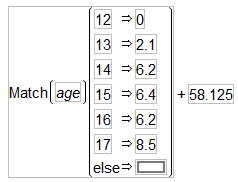The Match conditional evaluates faster than the If function because the age variable is evaluated only once for each row in the data table. The If condition must evaluate the age variable at each If clause for each row until a clause evaluates as true.